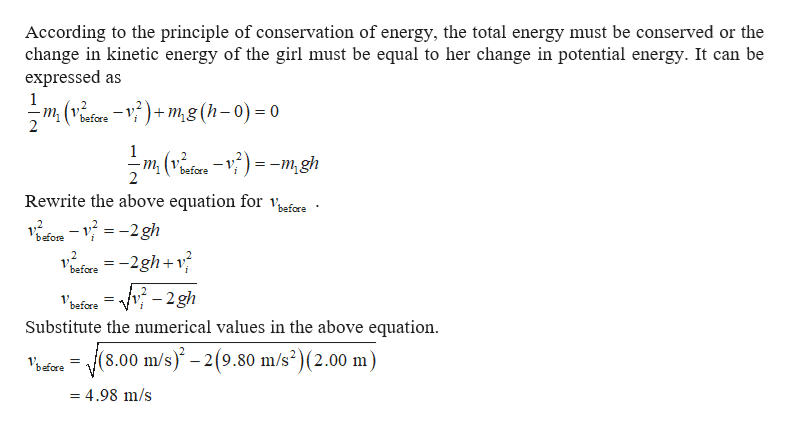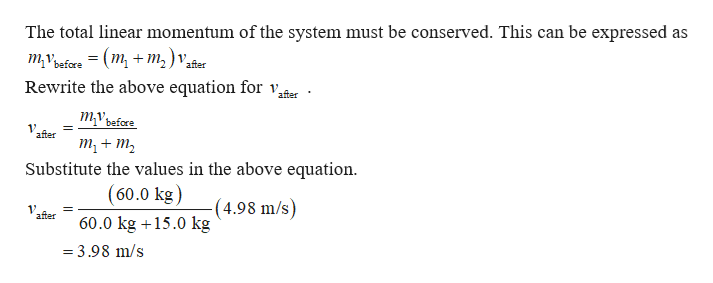# m2тyh

Question
81 views

A girl of mass m1=60.0 kilograms springs from a trampoline with an initial upward velocity of vi=8.00 meters per second. At height h=2.00 meters above the trampoline, the girl grabs a box of mass m2=15.0 kilograms. (Figure 1)

For this problem, use g=9.80 meters per second per second for the magnitude of the acceleration due to gravity.

What is the speed vbefore of the girl immediately before she grabs the box?

What is the speed vafter of the girl immediately after she grabs the box?

What is the maximum height hmax that the girl (with box) reaches? Measure hmax with respect to the top of the trampoline.
check_circle

Step 1help_outlineImage TranscriptioncloseAccording to the principle of conservation of energy, the total energy must be conserved or the change in kinetic energy of the girl must be equal to her change in potential energy. It can be expressed as 1 m (vefere -V)+m,g (h-0) = 0 1 -m, (v^efce-V)=-m, gh before 2 Rewrite the above equation for vafere before before = -2gh+v^ -v- 2gh i Vbafore = Substitute the numerical values in the above equation (8.00 m/s)-2(9.80 m/s2)(2.00 m) = 4.98 m/s fullscreen
Step 2help_outlineImage TranscriptioncloseThe total linear momentum of the system must be conserved. This can be expressed as тубre — (m, + т,) ,. Rewrite the above equation for v, after mvbafore 1 after т, + т, Substitute the values in the above equation (60.0 kg) (4.98 m/s) 1V after 60.0 kg15.0 kg = 3.98 m/s fullscreen

### Want to see the full answer?

See Solution

#### Want to see this answer and more?

Solutions are written by subject experts who are available 24/7. Questions are typically answered within 1 hour.*

See Solution
*Response times may vary by subject and question.
Tagged in

### Physics